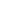Some test text!search
< Windows samples

# DOCX to PDF Conversion in C++

Sample C++ code to use PDFTron SDK for direct, high-quality Word to PDF conversion by using the pdftron.PDF.Convert utility class. This conversion is performed entirely within PDFTron SDK and has no external or system dependencies. Conversion results will be the same on all platforms. Learn more about our PDF Conversion Library.

Start Trial

Step 2: Add the code:

``````//------------------------------------------------------------------------------
// Consult legal.txt regarding legal and license information.
//------------------------------------------------------------------------------

#include <iostream>
#include <sstream>
#include <PDF/PDFNet.h>
#include <PDF/Convert.h>

//------------------------------------------------------------------------------
// The following sample illustrates how to use the PDF::Convert utility class
// to convert .docx files to PDF
//
// This conversion is performed entirely within the PDFNet and has *no*
// external or system dependencies dependencies -- Conversion results will be
// the same whether on Windows, Linux or Android.
//
//------------------------------------------------------------------------------

using namespace pdftron;
using namespace PDF;

UString input_path = "../../TestFiles/";
UString output_path = "../../TestFiles/Output/";

void SimpleDocxConvert(UString input_filename, UString output_filename)
{
// Start with a PDFDoc (the conversion destination)
PDFDoc pdfdoc;

// perform the conversion with no optional parameters
Convert::WordToPDF(pdfdoc, input_path + input_filename, NULL);

// save the result
pdfdoc.Save(output_path + output_filename, SDF::SDFDoc::e_linearized, NULL);

// And we're done!
std::cout << "Saved " << output_filename << std::endl;
}

void FlexibleDocxConvert(UString input_filename, UString output_filename)
{
// Start with a PDFDoc (the conversion destination)
PDFDoc pdfdoc;

WordToPDFOptions options;

// set up smart font substitutions to improve conversion results
// in situations where the original fonts are not available
options.SetSmartSubstitutionPluginPath(input_path);

// create a conversion object -- this sets things up but does not yet
// perform any conversion logic.
// in a multithreaded environment, this object can be used to monitor
// the conversion progress and potentially cancel it as well
DocumentConversion conversion = Convert::WordToPDFConversion(
pdfdoc, input_path + input_filename, &options);

// Print the progress of the conversion.
/*
std::cout << "Status: " << conversion.GetProgress()*100 << "%, "
<< conversion.GetProgressLabel() << std::endl;
*/

// actually perform the conversion
// this particular method will not throw on conversion failure, but will
// return an error status instead

while (conversion.GetConversionStatus() == DocumentConversion::eIncomplete)
{
conversion.ConvertNextPage();
// print out the progress status as we go
/*
std::cout << "Status: " << conversion.GetProgress()*100 << "%, "
<< conversion.GetProgressLabel() << std::endl;
*/
}

if(conversion.GetConversionStatus() == DocumentConversion::eSuccess)
{
int num_warnings = conversion.GetNumWarnings();

// print information about the conversion
for (int i = 0; i < num_warnings; ++i)
{
std::cout << "Conversion Warning: "
<< conversion.GetWarningString(i) << std::endl;
}

// save the result
pdfdoc.Save(output_path + output_filename, SDF::SDFDoc::e_linearized, NULL);
// done
std::cout << "Saved " << output_filename << std::endl;
}
else
{
std::cout << "Encountered an error during conversion: "
<< conversion.GetErrorString() << std::endl;
}

}

int main(int argc, char *argv[])
{
// The first step in every application using PDFNet is to initialize the
// library. The library is usually initialized only once, but calling
// Initialize() multiple times is also fine.
int ret = 0;

PDFNet::Initialize();
PDFNet::SetResourcesPath("../../../Resources");

try
{
// first the one-line conversion function
SimpleDocxConvert("simple-word_2007.docx", "simple-word_2007.pdf");

// then the more flexible line-by-line conversion API
FlexibleDocxConvert("the_rime_of_the_ancient_mariner.docx",
"the_rime_of_the_ancient_mariner.pdf");

// and one more for the road...
SimpleDocxConvert("wrap_poly_demo.docx", "wrap_poly_demo.pdf");

}
catch (Common::Exception& e)
{
std::cout << e << std::endl;
ret = 1;
}
catch (...)
{
std::cout << "Unknown Exception" << std::endl;
ret = 1;
}

PDFNet::Terminate();
std::cout << "Done.\n";
return ret;
}``````